# Advanced Reactors and Fuel Cycles Group

### Kathryn (Katy) Huff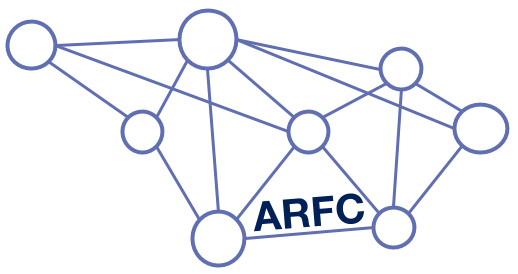## Insights at Disparate Scales## The Nuclear Fuel Cycle

Hundreds of discrete facilities mine, mill, convert, fabricate, transmute, recycle, and store nuclear material.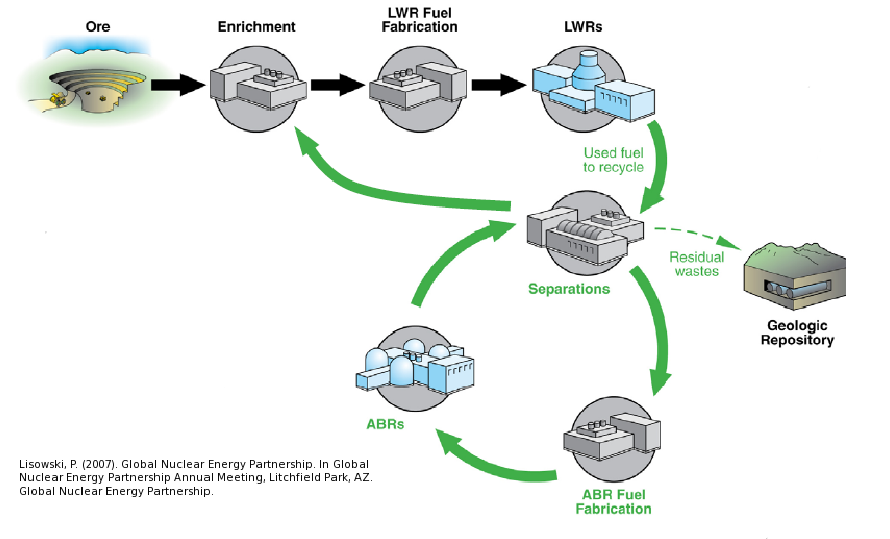## Fuel Cycle Metrics

• Mass Flow
• proliferation resistance and physical protection (PRPP) indices.
• Cost
• levelized cost of electricity,
• facility life cycle costs.
• Economics
• power production, facility deployments,
• dynamic pricing and feedback.
• Disruptions
• reliability, safety,
• system robustness.

## Agent Based Systems Analysis

A facility might create material.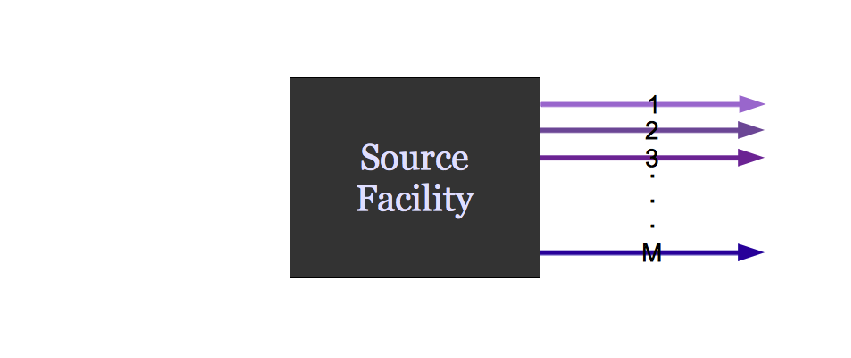## Agent Based Systems Analysis

It might request material.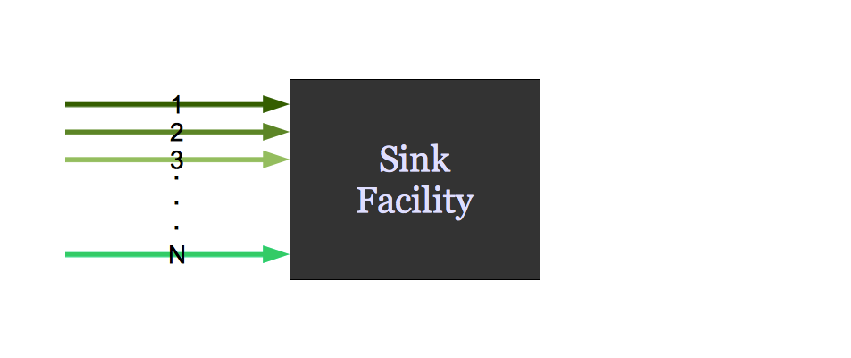## Agent Based Systems Analysis

It might do both.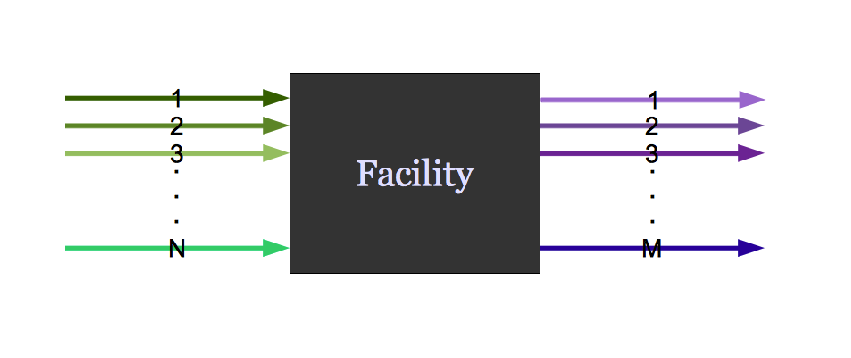## Agent Based Systems Analysis

Even simple fuel cycles have many independent agents.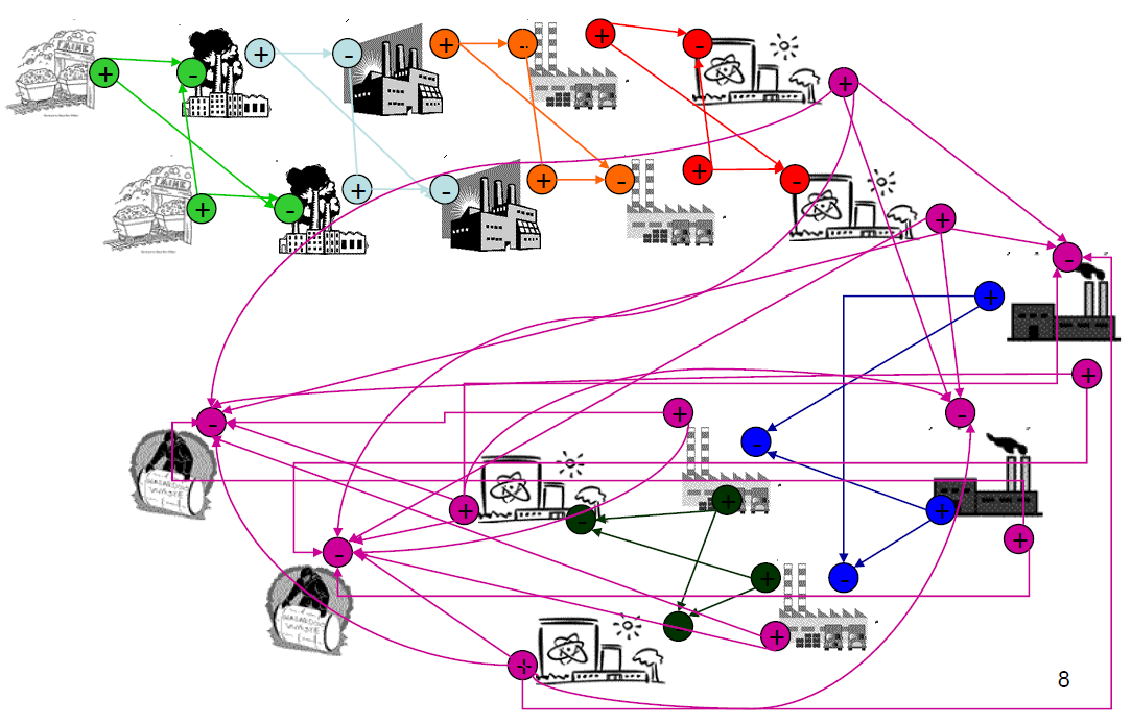## Dynamic Resource Exchange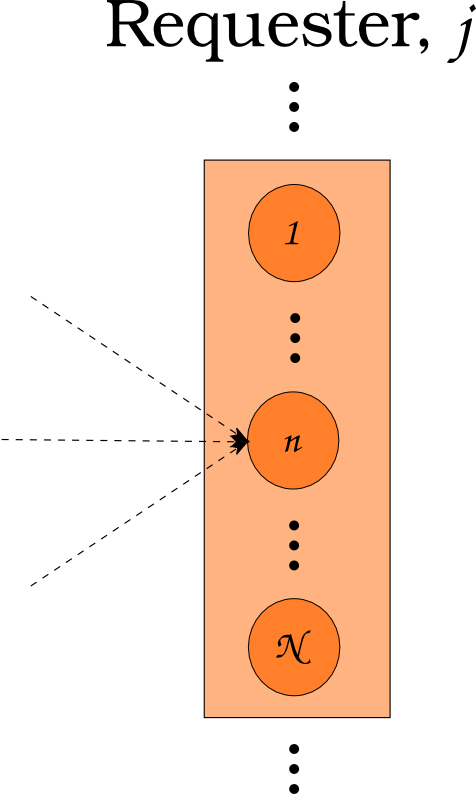$N_i \subset N$

## Dynamic Resource Exchange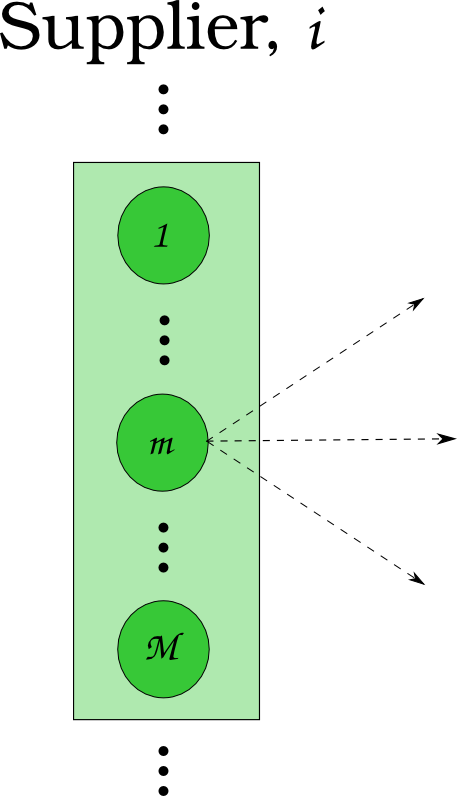$N_j \subset N$

## Dynamic Resource Exchange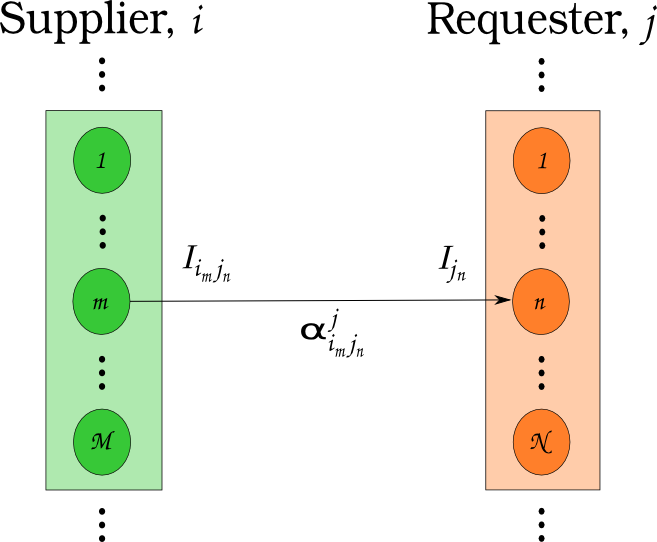$N_i \cup N_j = N$

## Transition Analysis

• LWR to SFR
• $T_0 = 2015$
• $T_f <= 2215$
• $C_0 = 100$ GWe LWR
• Annual nuclear energy demand growth: 1%
• Spent LWR fuel reprocessed to fabricate FR fuel
• Spent FR fuel reprocessed to fabricate FR fuel

## Transition Analysis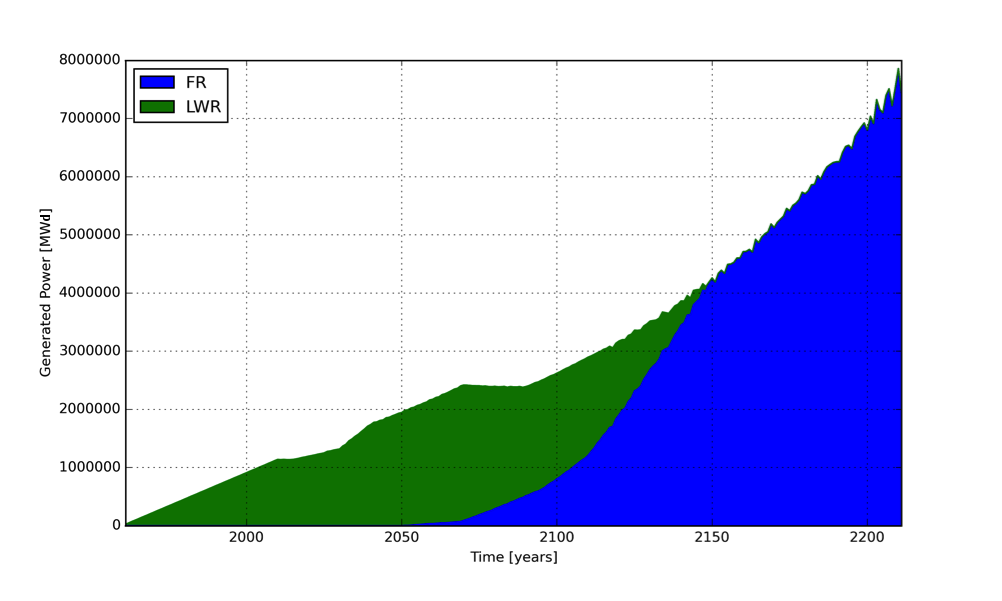Power generated by reactor type.

## Transition Analysis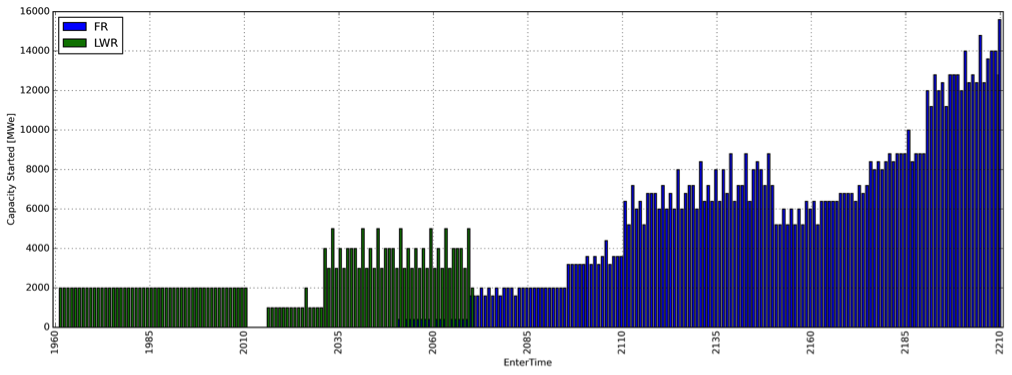Capacity deployed each year, by reactor type.## Moltres (coupling in MOOSE)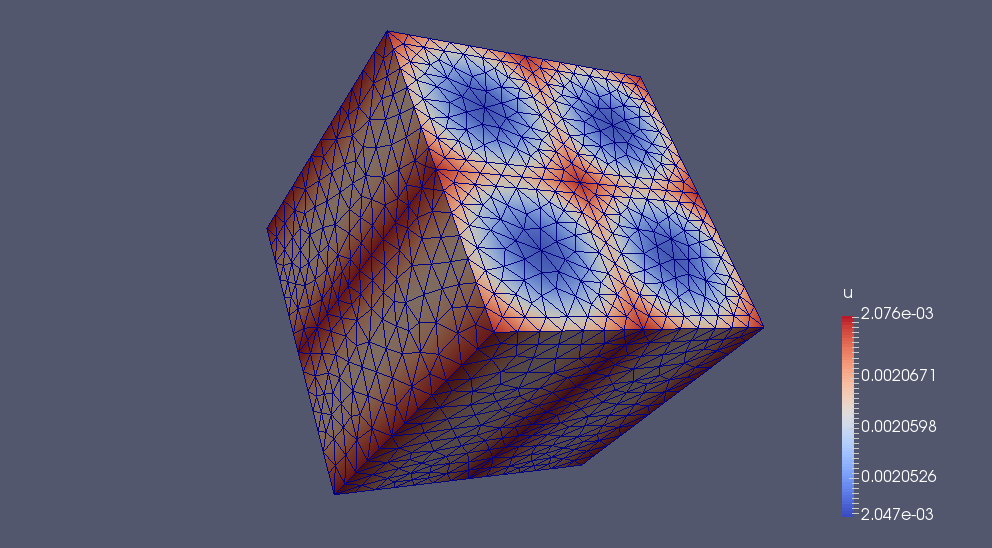Alexander Lindsay, 2016

## Moltres (data via Serpent)Alexander Lindsay, 2016

## Reactor Physics and Serpent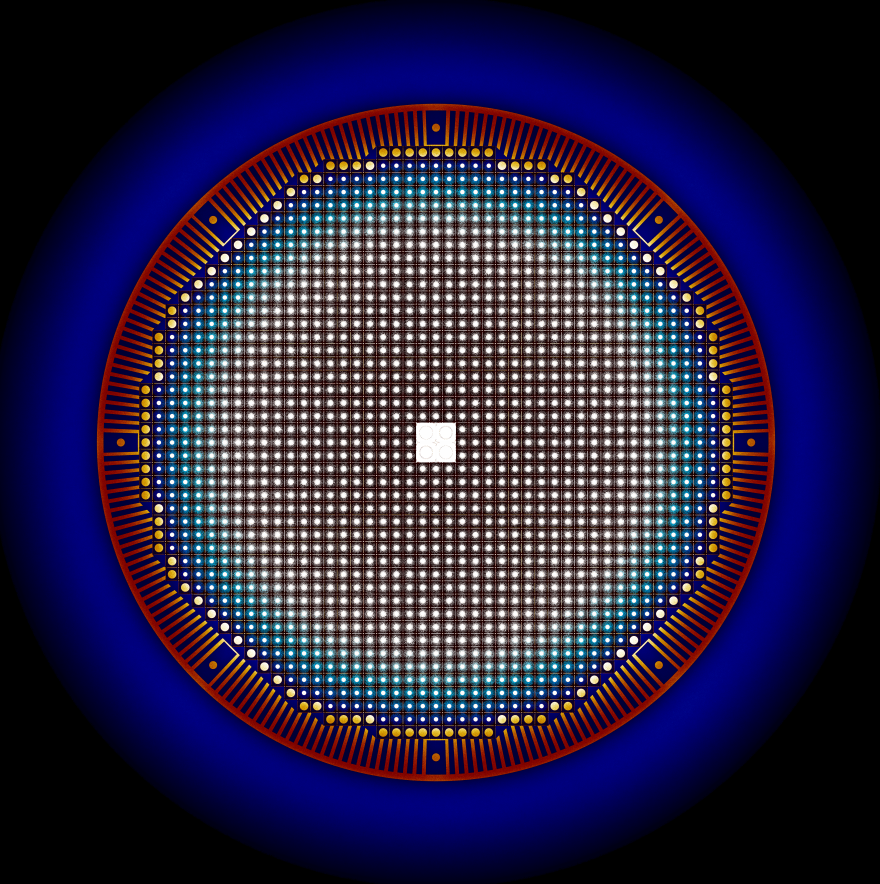Andrei Rykhlevskii, 2017

## Reactor Physics and Serpent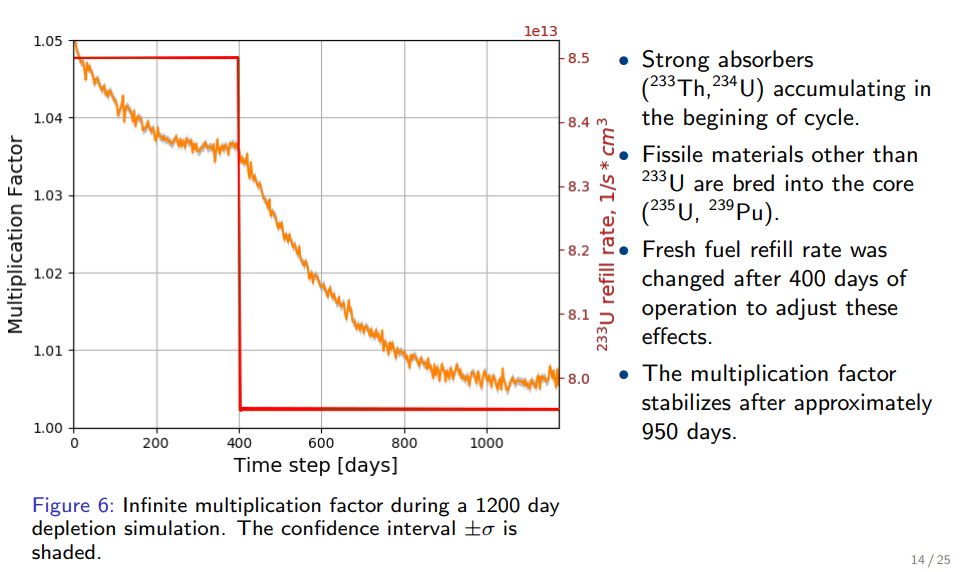Andrei Rykhlevskii, 2017

## Reactor Physics and Serpent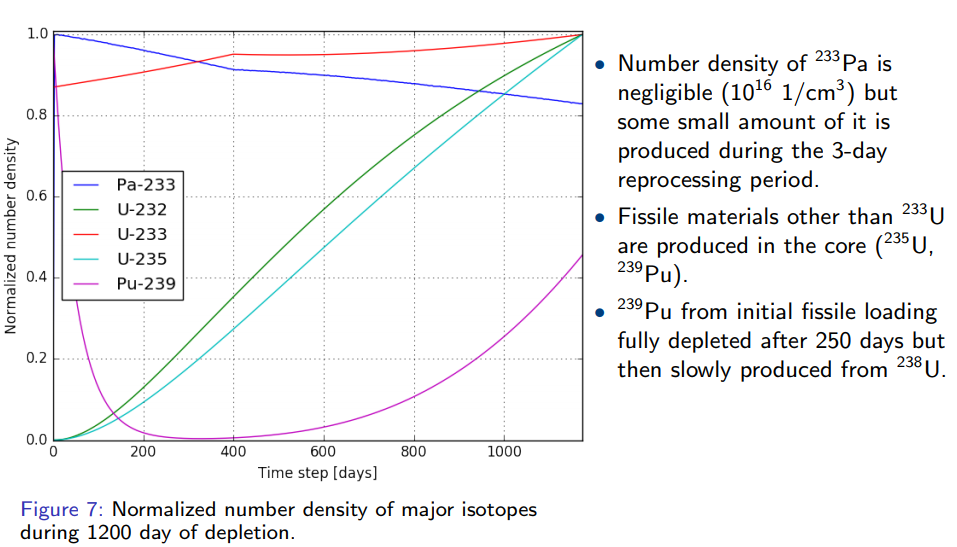Andrei Rykhlevskii, 2017

## Simulation Methods

• Monte Carlo Methods
• Deterministic Methods
• Hybrid Methods

## Application Specific Data Processing

• Energy discretization
• multigroup
• pointwise
• piecewise linear continuous
• Resonance integration
• ...PyRK: Python for Reactor Kinetics

## Review of Nuclear Reactor Kinetics$\sigma(E,\vec{r},\hat{\Omega},T,x,i)$$k=1$

## Reactivity

\begin{align} k &= \mbox{"neutron multiplication factor"}\\ &= \frac{\mbox{neutrons causing fission}}{\mbox{neutrons produced by fission}}\\ \rho &= \frac{k-1}{k}\\ \rho &= \mbox{reactivity}\\ \end{align}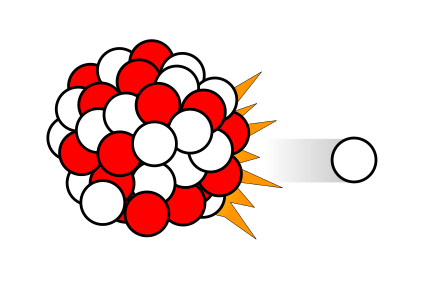$\beta_i, \lambda_{d,i}$

### Ramp Reactivity Insertion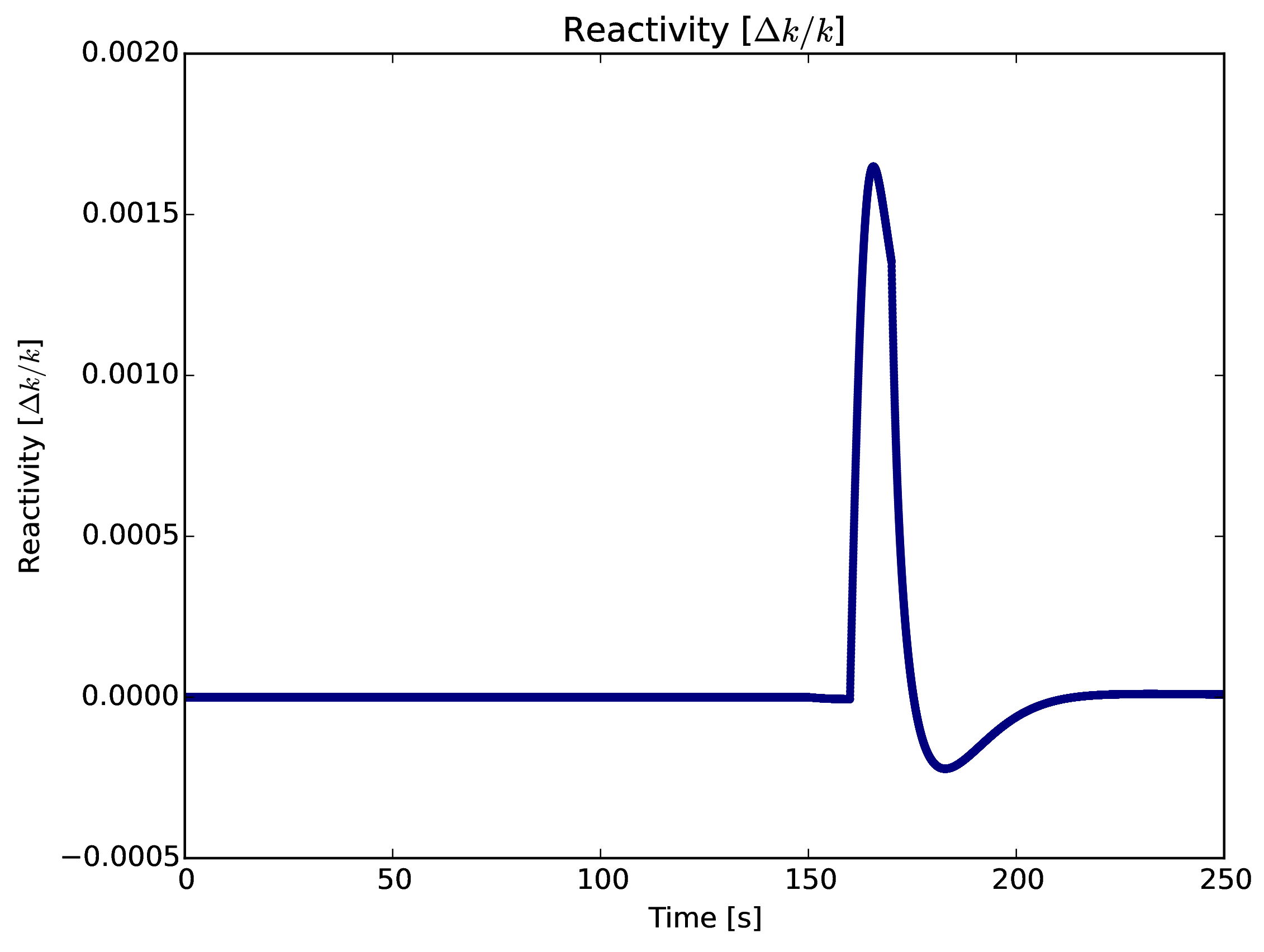### Fuel Layer Temperature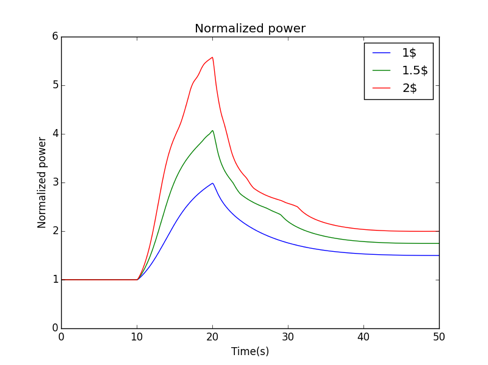### Coolant Temperature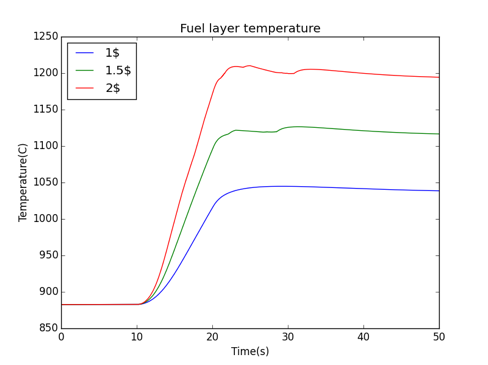## A Few of My Favorite Things

• C++, Python, Fortran
• Serpent, MOOSE, ORIGEN
• xml, markdown, rst, $\LaTeX$
• Doxygen, sphinx
• CMake, conda, macports